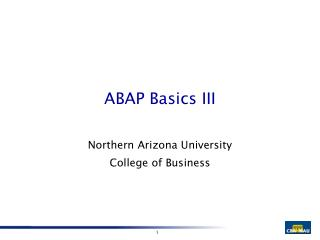DownloadDownload PresentationABAP Basics III

# ABAP Basics III

Télécharger la présentation## ABAP Basics III

- - - - - - - - - - - - - - - - - - - - - - - - - - - E N D - - - - - - - - - - - - - - - - - - - - - - - - - - -
##### Presentation Transcript

1. ABAP Basics III Northern Arizona University College of Business

2. Types • It is possible to define new data types. • These are known as user-defined types. • New types are defined using the Types statement.

3. Types • The syntax is identical to the Data statement.

4. Types Types: Bank_Acct(28) Type C. Data: Ckecking_Acct Type Bank_Acct. Savings_Acct Type Bank_Acct.

5. Types Types Begin of Demo_Type , D_ID Type I , D_Name(20) Type C , End of Demo_Type . Data: Old_Demo Type Demo_Type , New_Demo Type Demo_Type .

6. Formatting Format Intensified . Write ‘Whatever’ . Format Reset .

7. Formatting Format Inverse . Write ‘Whatever’ . Format Reset .

8. Formatting Format Color 3 . “ 0-7 Write ‘Whatever’ . Format Reset .

9. Arithmetic Operators + add - Subtract * multiply / divide ** exponential Div integer quotient of division Mod integer remainder of division

10. Compute Statement Compute Result = A + B . Result = A + B . Result = (A + B) / C .

11. Add Statement Add 1 to Counter . or Counter = Counter + 1 . Add F1 then F2 until F9 giving Result .

12. Character Data – Offset & Length Data: F1(6) Type C value ‘abcdef’ . F2(6) Type C value ‘ ‘ . Move ‘x’ to F1+2 . “will space fill Move ‘x’ to F1+2(1) . “no space fill Move F1+0(3) to F2 .

13. Character Data – Shift Data: F1(6) Type C value ‘abcdef’ . Shift F1 . “shifts left Shift F1 circular . Shift F1 right . Shift F1 by 4 places .

14. Character Data – Replace Data: F1(7) Type C value ‘XXX-NAU’ . Replace ‘XXX’ with ‘CBA’ into F1 . • The strings are case sensitive .

15. Character Data – Search Data: F1(7) Type C value ‘CBA-NAU’ . Search F1 for‘CBA*’ . • Sy-subrc = 0 (match) or 4 (no match).

16. Case Statement • The Case statement is used when there is a given set of values in a field .

17. Case Statement Data Field1 Type I . Case Field1 . When 1 . Write ‘The value is 1’ . When 2 . Write ‘The value is 2’ . When Others . Write ‘The value is greater than 2’ . EndCase .

18. Case Statement Data Field1(1) Type C . Case Field1 . When ‘a’ . Write ‘The value is a’ . When ‘b’ . Write ‘The value is b’ . When Others . Write ‘The value is greater than b’ . EndCase .

19. Case Statement Case sy-subrc . When 0 . Write ‘The value is zero’ . When Others . Write ‘The value is not zero’ . EndCase .

20. If Statement If A = B . Whatever = 0 . EndIf .

21. If Statement If A = B . Whatever = 0 . Else . Whatever = 1 . EndIf .

22. If Statement If A < 50 . Whatever = 0 . ElseIf A = 50 . Whatever = 1 . Else . Whatever = 2 . EndIf .

23. Logical Operators > GT < LT >= GE => <= LE =< = EQ <> NE ><

24. Logical Operators Is Initial Between value1 And value 2 CO contains only CA contains any CS contains string CP contains pattern

25. If Statement If W_Field is Initial . If Not ( W_Field is Initial ) . If W_Field is between 10 and 20 .

26. If Statement Data W_Field(6) type C Value ‘abcdef’ . If W_Field CO ‘abcdef’ . “only If W_Field CA ‘a’ . “any IF W_Field CS ‘bc’ . “string IF W_Field CP ‘*b+d*’ “pattern

27. Looping • Do Loop • While Loop • Loop

28. Do Loop • Used when a fixed number of iterations is known. Do 10 times. . . . . . EndDo.

29. Do Loop Data Cnt type I value 10 . Do Cnt times. Write: / ‘the loop count is: ‘, sy-index . EndDo.

30. Sy-Index • Sy-Index automatically stores the loop pass number.

31. Do Loop - Exit Data Cnt type I value 10 . Do Cnt times. Write: / ‘the loop count is: ‘, sy-index . If sy-index = 5 . Exit . EndIf . EndDo.

32. While Loop Data: Cnt type I value 0 , Limit type I value 10 . While Cnt < Limit . Cnt = Cnt + 1 . Write: / ‘the loop count is: ‘, Cnt . EndWhile .

33. Loop • The Loop statement is used with internal tables. Loop . . . . . . EndLoop .